# Quesnel Exploitation Analysis 2016

The suggested citation for this analytic report is:

Thorley, J.L. and Dalgarno, S. (2017) Quesnel Exploitation Analysis 2016. A Poisson Consulting Analysis Report. URL: http://www.poissonconsulting.ca/f/986075165.

## Background

Quesnel Lake supports a recreational fishery for large Bull Trout, Lake Trout and Rainbow Trout. To provide information on the natural and fishing mortality, trout were caught by angling and tagged with acoustic transmitters and/or reward tags.

## Methods

### Data Preparation

The outing, receiver deployment and fish capture and recapture information were provided by the Ministry of Forests, Lands and Natural Resource Operations and entered by Vicky Lipinski and databased by Gary Pavan.

The data were prepared for analysis using R version 3.4.0 (R Core Team 2017). Receivers were assumed to have a detection range of 500 m. Only individuals with a fork length (FL) $$\geq$$ 450 mm, an acoustic tag life $$\geq$$ 365 days (if acoustically tagged) and a $100 and$10 reward tags were included in the survival analysis.

### Data Analysis

Hierarchical Bayesian models were fitted to the data using R version 3.4.0 (R Core Team 2017) and JAGS 4.2.0 (Plummer 2015) which interfaced with each other via the jmbr package. For additional information on hierarchical Bayesian modelling in the BUGS language, of which JAGS uses a dialect, the reader is referred to Kery and Schaub (2011, 41–44).

Unless indicated otherwise, the models used prior distributions that were vague in the sense that they did not affect the posterior distributions (Kery and Schaub 2011, 36). The posterior distributions were estimated from 2,000 Markov Chain Monte Carlo (MCMC) samples thinned from the second halves of four chains (Kery and Schaub 2011, 38–40). Model convergence was confirmed by ensuring that $$\hat{R} < 1.1$$ (Kery and Schaub 2011, 40) for each of the monitored parameters in the model(Kery and Schaub 2011, 61).

The posterior distributions of the fixed (Kery and Schaub 2011, 75) parameters are summarised in terms of the point estimate, standard deviation (sd), the z-score, lower and upper 95% confidence/credible limits (CLs) and the p-value (Kery and Schaub 2011, 37, 42). The estimate is the median (50th percentile) of the MCMC samples, the z-score is $$\mathrm{mean}/\mathrm{sd}$$ and the 95% CLs are the 2.5th and 97.5th percentiles. A p-value of 0.05 indicates that the lower or upper 95% CL is 0.

The results are displayed graphically by plotting the modeled relationships between particular variables and the response with the remaining variables held constant. In general, continuous and discrete fixed variables are held constant at their mean and first level values respectively while random variables are held constant at their typical values (expected values of the underlying hyperdistributions) (Kery and Schaub 2011, 77–82). Where informative the influence of particular variables is expressed in terms of the effect size (i.e., percent change in the response variable) with 95% CIs (Bradford, Korman, and Higgins 2005).

### Model Descriptions

#### Survival

The natural mortality and exploitation were estimated using a Bayesian individual state-space survival model (Thorley and Andrusak 2017) with monthly intervals. The survival model incorporated natural and handling mortality, acoustic detection, inter-section movement, T-bar tag loss, recapture and reporting. In addition to assumptions 1 to 10, in Thorley and Andrusak (2017), the model also assumes that:

• The effect of handling on mortality lasts up to two months after capture.
• Annual T-bar tag-loss is between 1 and 30%.
• All recaptured fish are retained.
• Reporting of recaptured fish with one or more T-bar tags is between 90 and 100%.

#### Condition

The expected weight of fish of a given length were estimated from the data using a mass-length model (He et al. 2008). Key assumptions of the condition model include:

• The expected weight is allowed to vary with length and date.
• The residual variation in weight is log-normally distributed.

#### Yield-Per-Recruit

The optimal fishing mortality (to maximize number of individuals harvested) was calculated using a yield-per-recruit approach (Bison, O’Brien, and Martell 2003). Key assumptions include:

• The population is at equilibrium.
• All captured individuals are retained.
• There are no Allee effects.
• The life-history parameters are fixed.

## Results

### Survival

model{
bMortality ~ dnorm(-3, 3^-2)
bMortalityHandling ~ dnorm(0, 2^-2)
bTagLoss ~ dunif(1 - (1 - 0.01)^(1/12), 1 - (1 - 0.30)^(1/12))
bDetected ~ dunif(0, 1)
bMoved ~ dunif(0, 1)
bRecaptured ~ dunif(0, 1 - (1 - 0.50)^(1/12))
bReported ~ dunif(0.9, 1.00)

for (i in 1:nCapture){
logit(eMortality[i,PeriodCapture[i]]) <- bMortality + bMortalityHandling
eTagLoss[i,PeriodCapture[i]] <- bTagLoss
eDetected[i,PeriodCapture[i]] <- bDetected
eMoved[i,PeriodCapture[i]] <- bMoved
eRecaptured[i,PeriodCapture[i]] <- bRecaptured
eReported[i,PeriodCapture[i]] <- bReported

InLake[i,PeriodCapture[i]] <- 1
Alive[i,PeriodCapture[i]] ~ dbern(1-eMortality[i,PeriodCapture[i]])

TBarTag100[i,PeriodCapture[i]] ~ dbern(1-eTagLoss[i,PeriodCapture[i]])
TBarTag10[i,PeriodCapture[i]] ~ dbern(1-eTagLoss[i,PeriodCapture[i]])

Detected[i,PeriodCapture[i]] ~ dbern(Monitored[i,PeriodCapture[i]] * eDetected[i,PeriodCapture[i]])
Moved[i,PeriodCapture[i]] ~ dbern(Alive[i,PeriodCapture[i]] * Monitored[i,PeriodCapture[i]] * eMoved[i,PeriodCapture[i]])

Recaptured[i,PeriodCapture[i]] ~ dbern(Alive[i,PeriodCapture[i]] * eRecaptured[i,PeriodCapture[i]])
Reported[i,PeriodCapture[i]] ~ dbern(Recaptured[i,PeriodCapture[i]] * eReported[i,PeriodCapture[i]] * step(TBarTag100[i,PeriodCapture[i]] + TBarTag10[i,PeriodCapture[i]] - 1))

for(j in (PeriodCapture[i]+1):nPeriod) {
logit(eMortality[i,j]) <- bMortality + bMortalityHandling * step(PeriodCapture[i] - j + 2)
eTagLoss[i,j] <- bTagLoss
eDetected[i,j] <- bDetected
eMoved[i,j] <- bMoved
eRecaptured[i,j] <- bRecaptured
eReported[i,j] <- bReported

InLake[i,j] ~ dbern(InLake[i,j-1] * (1-Recaptured[i,j-1]))
Alive[i,j] ~ dbern(Alive[i,j-1] * (1-Recaptured[i,j-1]) * (1-eMortality[i,j-1]))

TBarTag100[i,j] ~ dbern(TBarTag100[i,j-1] * (1-Recaptured[i,j-1]) * (1-eTagLoss[i,j]))
TBarTag10[i,j] ~ dbern(TBarTag10[i,j-1] * (1-Recaptured[i,j-1]) * (1-eTagLoss[i,j]))

Detected[i,j] ~ dbern(InLake[i,j] * Monitored[i,j] * eDetected[i,j])
Moved[i,j] ~ dbern(Alive[i,j] * Monitored[i,j] * eMoved[i,j])

Recaptured[i,j] ~ dbern(Alive[i,j] * eRecaptured[i,j])

Reported[i,j] ~ dbern(Recaptured[i,j] * eReported[i,j] * step(TBarTag100[i,j] + TBarTag10[i,j] - 1))
}
}
..

Template 1.

### Condition

model {
bWeight ~ dnorm(0, 2^-2)
bWeightLength ~ dnorm(3, 2^-2)
bWeightDayte ~ dnorm(0, 2^-2)

sWeight ~ dnorm(0, 5^-2)
for(i in 1:length(Length)) {
eWeight[i] <- bWeight + bWeightDayte * Dayte[i] + bWeightLength * Length[i]
Weight[i] ~ dlnorm(eWeight[i], exp(sWeight)^-2)
}
W_p <- bWeightLength
W_500 <- exp(bWeight)
..

Template 2.

### Survival

Table 1. Parameter descriptions.

Parameter Description
bDetected Logit monthly probability of detection if in-lake
bMortality Logit monthly probability of dying of natural causes
bMortalityHandling Effect of capture and handling on bMortality
bMoved Logit monthly probability of being detected moving between sections if alive
bRecaptured Logit monthly probability of being recaptured
bReported Monthly probability of being reported if recaptured with one or more T-bar tags
bTagLoss Monthly probability of loss for a single T-bar tag

#### Bull Trout

Table 2. Model coefficients.

term estimate sd zscore lower upper pvalue
bDetected 0.4868612 0.0127726 38.1179606 0.4608447 0.5120932 0.0005
bMortality -3.7658797 0.3687714 -10.3231177 -4.6247301 -3.1941157 0.0005
bMortalityHandling 0.7427651 0.8354063 0.7850448 -1.2458831 1.9908157 0.3210
bMoved 0.1730442 0.0135304 12.7720001 0.1463824 0.1985949 0.0005
bRecaptured 0.0030820 0.0016779 1.9997871 0.0009506 0.0072446 0.0005
bReported 0.9528007 0.0285138 33.3773691 0.9029412 0.9973418 0.0005
bTagLoss 0.0139901 0.0069068 2.1003925 0.0033015 0.0277869 0.0005

Table 3. Model summary.

n K nsamples nchains nsims duration rhat converged
3225 7 2000 4 512000 3021.74316549301s (~50.36 minutes) 1.1 TRUE

#### Lake Trout

Table 4. Model coefficients.

term estimate sd zscore lower upper pvalue
bDetected 0.7990457 0.0075669 105.610228 0.7844283 0.8136332 5e-04
bMortality -4.0586397 0.2186842 -18.594192 -4.5125622 -3.6695118 5e-04
bMortalityHandling 1.4965586 0.3038667 4.965026 0.9165637 2.1078007 5e-04
bMoved 0.4543795 0.0116755 38.935016 0.4318811 0.4776996 5e-04
bRecaptured 0.0031017 0.0006867 4.617492 0.0019751 0.0047379 5e-04
bReported 0.9719873 0.0262259 36.831189 0.9065763 0.9990426 5e-04
bTagLoss 0.0023166 0.0019086 1.491938 0.0008957 0.0081360 5e-04

Table 5. Model summary.

n K nsamples nchains nsims duration rhat converged
17888 7 2000 4 256000 17573.3580644131s (~4.88 hours) 1.15 FALSE

#### Rainbow Trout

Table 6. Model coefficients.

term estimate sd zscore lower upper pvalue
bDetected 0.5768390 0.0085915 67.148782 0.5590583 0.5941297 0.0005
bMortality -2.7595617 0.1257838 -21.950013 -3.0099894 -2.5177111 0.0005
bMortalityHandling -0.2650639 0.2370517 -1.115263 -0.7320028 0.2060014 0.2470
bMoved 0.7123151 0.0112610 63.251258 0.6903444 0.7345269 0.0005
bRecaptured 0.0143381 0.0023400 6.191881 0.0103606 0.0191715 0.0005
bReported 0.9820828 0.0210545 46.337667 0.9225844 0.9992623 0.0005
bTagLoss 0.0045957 0.0024720 2.063455 0.0017078 0.0111733 0.0005

Table 7. Model summary.

n K nsamples nchains nsims duration rhat converged
13072 7 2000 4 512000 13649.9661698341s (~3.79 hours) 1 TRUE

### Condition

Table 8. Parameter descriptions.

Parameter Description
bWeight Intercept of eWeight
bWeightDayte Effect of Dayte on bWeight
bWeightLength Intercept of effect of Length on bWeight
Dayte Standardised day of year of capture
eWeight Log expected Weight
Length Log-transformed and centered on 500mm fork length
sWeight Log standard deviation of residual variation in log Weight
W500 Weight at 500 mm (kg)
Weight Mass (kg)
Wp Weight power term

#### Bull Trout

Table 9. Model coefficients.

term estimate sd zscore lower upper pvalue
bWeight 0.2728111 0.0261034 10.4163964 0.2198568 0.3232766 0.0005
bWeightDayte -0.0033242 0.0153712 -0.1937845 -0.0322424 0.0274966 0.8380
bWeightLength 3.3878190 0.1187208 28.5634413 3.1613642 3.6321259 0.0005
sWeight -2.2852314 0.1019954 -22.3751771 -2.4750712 -2.0805239 0.0005

Table 10. Derived coefficients.

term estimate sd zscore lower upper pvalue
W_p 3.387819 0.1187208 28.56344 3.161364 3.632126 5e-04
W_500 1.313652 0.0342298 38.35567 1.245898 1.381647 5e-04

Table 11. Model summary.

n K nsamples nchains nsims duration rhat converged
48 4 2000 4 4000 0.622170925140381s 1.01 TRUE

#### Lake Trout

Table 12. Model coefficients.

term estimate sd zscore lower upper pvalue
bWeight 0.3002094 0.0171114 17.576434 0.2681501 0.3355457 5e-04
bWeightDayte 0.0344538 0.0084407 4.061973 0.0179892 0.0506157 5e-04
bWeightLength 3.1415447 0.0770847 40.725147 2.9867524 3.2898791 5e-04
sWeight -2.0103260 0.0442248 -45.455845 -2.0944586 -1.9252312 5e-04

Table 13. Derived coefficients.

term estimate sd zscore lower upper pvalue
W_p 3.141545 0.0770847 40.72515 2.986752 3.289879 5e-04
W_500 1.350142 0.0231384 58.39137 1.307543 1.398703 5e-04

Table 14. Model summary.

n K nsamples nchains nsims duration rhat converged
245 4 2000 4 4000 0.96141791343689s 1.04 TRUE

#### Rainbow Trout

Table 15. Model coefficients.

term estimate sd zscore lower upper pvalue
bWeight 0.4455196 0.0185210 24.083905 0.4121099 0.4827810 5e-04
bWeightDayte 0.0732841 0.0127086 5.785053 0.0497775 0.0989143 5e-04
bWeightLength 3.0400317 0.0923604 32.890587 2.8488548 3.2060823 5e-04
sWeight -1.7038010 0.0462766 -36.811105 -1.7967906 -1.6141041 5e-04

Table 16. Derived coefficients.

term estimate sd zscore lower upper pvalue
W_p 3.040032 0.0923604 32.89059 2.848855 3.206082 5e-04
W_500 1.561301 0.0289797 53.91400 1.510000 1.620575 5e-04

Table 17. Model summary.

n K nsamples nchains nsims duration rhat converged
214 4 2000 4 4000 0.952297925949097s 1.01 TRUE

### Yield-Per-Recruit

Table 18. Assumed life-history parameters values in yield-per-recruit calculation by trout species.

Parameter Symbol Bull Lake Rainbow
Asymptotic length (mm) $$L_{\infty}$$ 1000.00 1000.00 1000.0
Growth constant (y-1) $$k$$ 0.13 0.15 0.2
Weight at 500 mm (kg) $$W_{500}$$ 1.30 1.40 1.6
Weight power $$W_{p}$$ 3.40 3.10 3.0
Length at maturity (mm) $$L_{m}$$ 500.00 500.00 500.0
Length at which 50% vulnerable to angling (mm) $$L_{v}$$ 400.00 400.00 400.0
Vulnerability power $$V_{p}$$ 10.00 10.00 10.0
Maximum annual reproductive rate $$R_{k}$$ 3.80 2.50 6.0

Table 19. Yield-per-recruit calculations.

Parameter Symbol Relationship
Annual interval fishing mortality rate $$U$$ $$\text{Optimized by yield-per-recruit analysis}$$
Annual interval natural mortality rate $$V$$ $$\text{From survival analysis}$$
Annual instantaneous fishing mortality rate $$F$$ $$-\text{log}(1-U)$$
Annual instantaneous natural mortality rate $$M$$ $$-\text{log}(1-V)$$
Length at age a (mm) $$L_{a}$$ $$L_{\infty}(1-\exp(-k \cdot a))$$
Weight at age a (kg) $$W_{a}$$ $$(W_{500} / 500^{W_p}) \cdot L_{a}^{W_p}$$
Relative fecundity at age a $$F_{a}$$ $$W_{a} \: \text{if} \: (L_{a} \geq L_{m}) \: \mathrm{otherwise}\: 0$$
Vulnerability to fishing at age a $$V_{a}$$ $$L_{a}^{V_p}/(L_{v}^{V_p} + L_{a}^{V_p})$$
Unfished survivorship to age a $$\lambda_{a}$$ $$\exp(-M)^{a-1}$$
Fished survivorship to age a $$\lambda'_{a}$$ $$\exp(-M+F \cdot V_a)^{a-1}$$
Unfished relative fecundity per age-1 recruit $$\phi_{0}$$ $$\sum(\lambda_{a} \cdot F_{a})$$
Fished relative fecundity per age-1 recruit $$\phi'_{0}$$ $$\sum(\lambda'_{a} \cdot F_{a})$$
Unfished age-1 recruits as percent of total unfished fish population (TUFP) (%) $$R_{0}$$ $$100/\sum\lambda_{a}$$
Stock productivity at low density $$\alpha$$ $$R_{k} \cdot \phi^{-1}$$
Stock density dependence $$\beta$$ $$(R_{k} - 1)/(R_{0} \cdot \phi)$$
Fished age-1 recruits as percent of TUFP (%) $$R'_{0}$$ $$(\alpha \cdot \phi'_{0} - 1)/(\beta \cdot \phi'_{0})$$
Equilibrium yield as percent of TUFP (%) $$Y_{e}$$ $$U \cdot R'_{0} \cdot \sum(\lambda'_{a} \cdot V_{a})$$

### Capture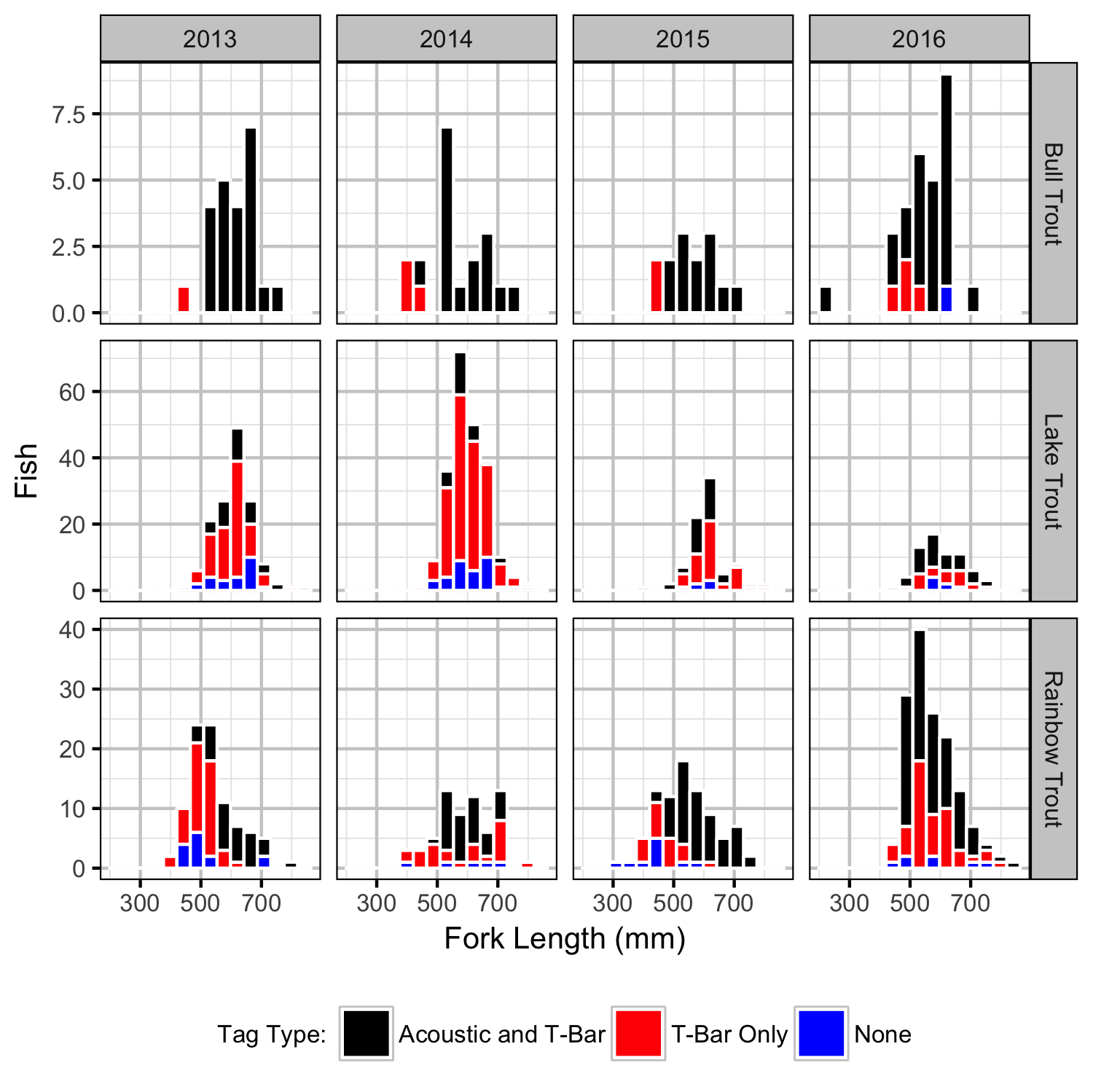Figure 1. Captures by fork length, year, species and tag type.

### Sections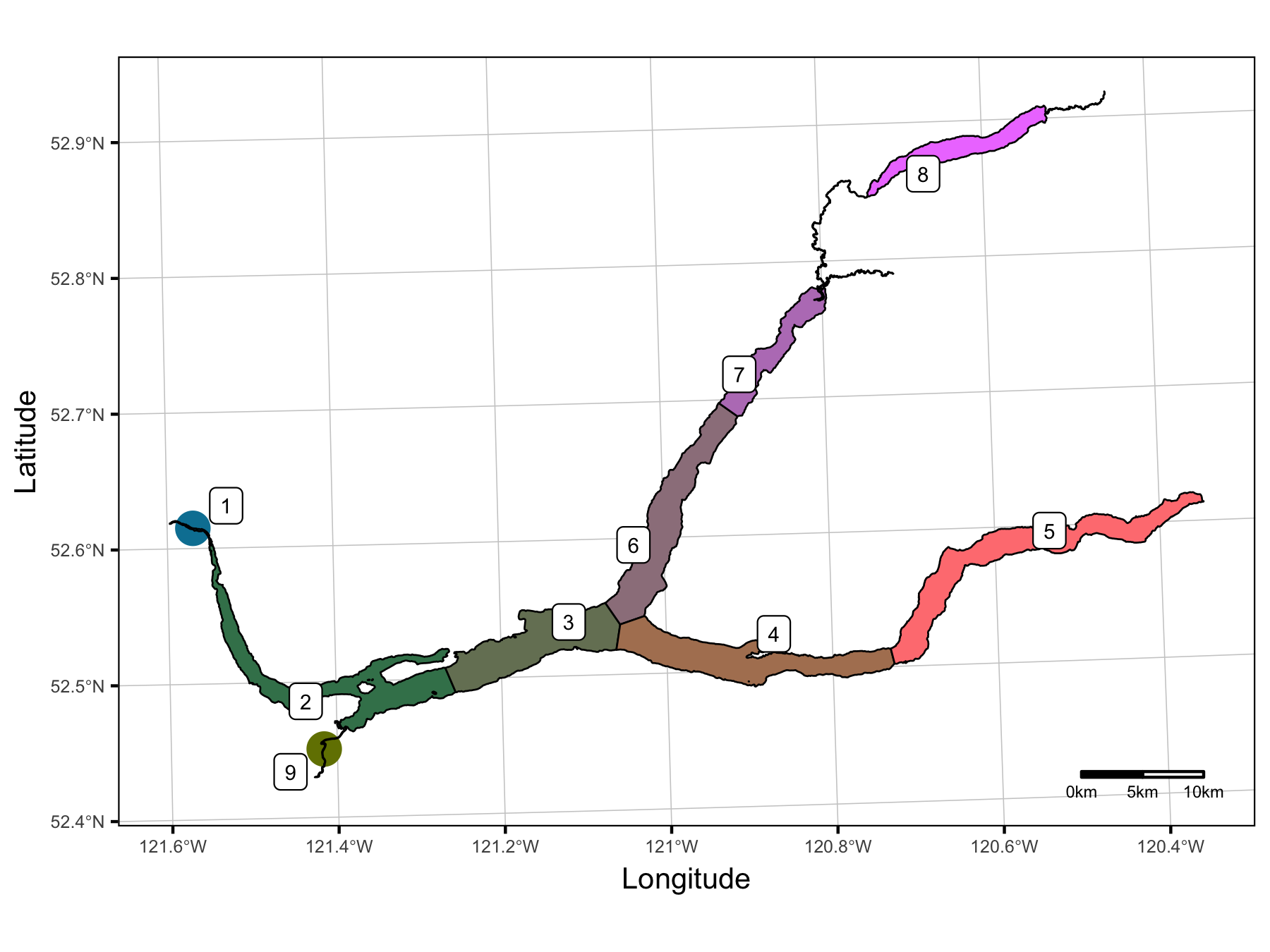Figure 2. Quesnel Lake sections. Color code is used to identify sections throughout analysis.

### Detections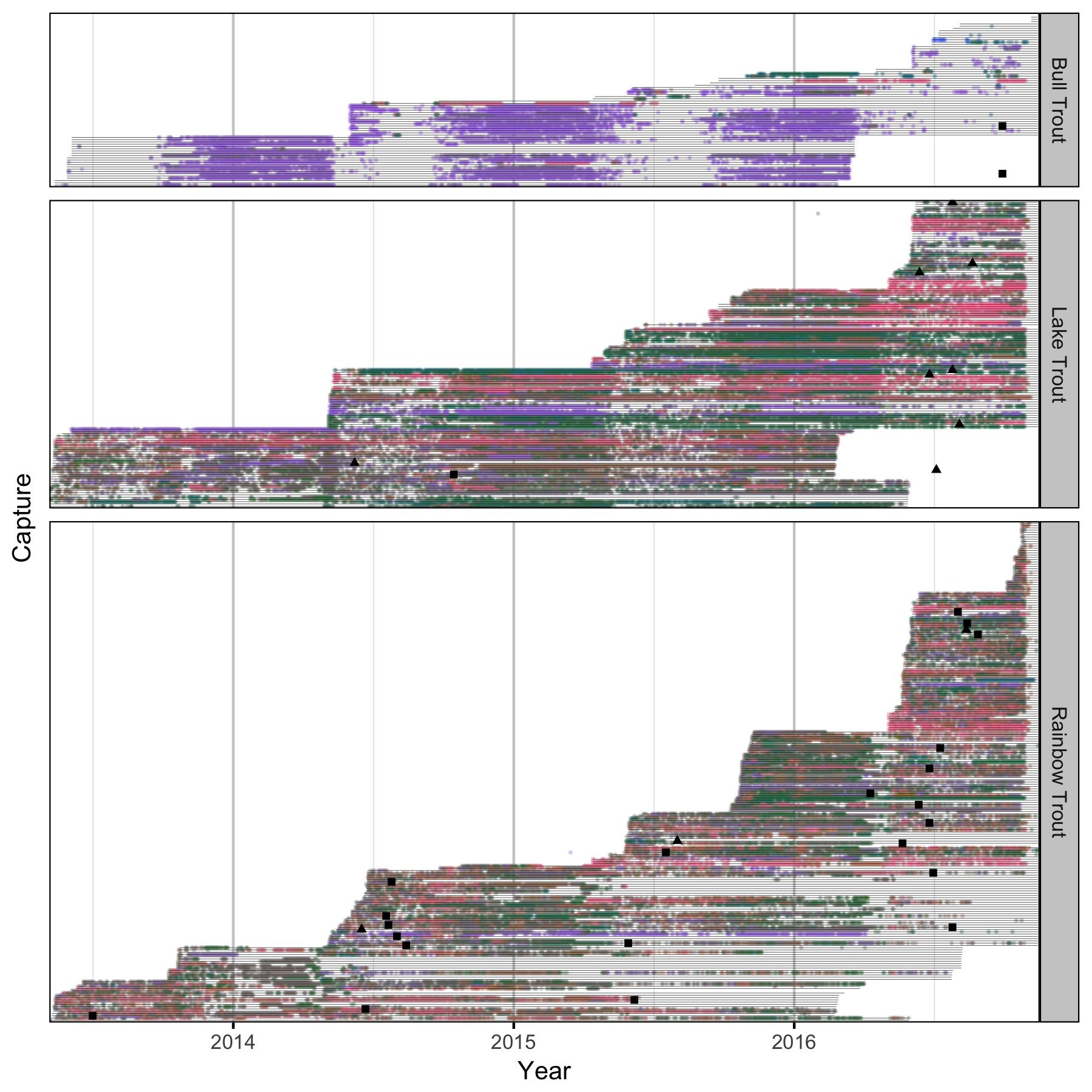Figure 4. Location (color) and date of detections by species for each acoustic tagged fish. Grey segments indicate estimated tag life from capture date. Black shapes indicate recapture date (square indicates that fish was not released; triangle indicates release).

### Section Use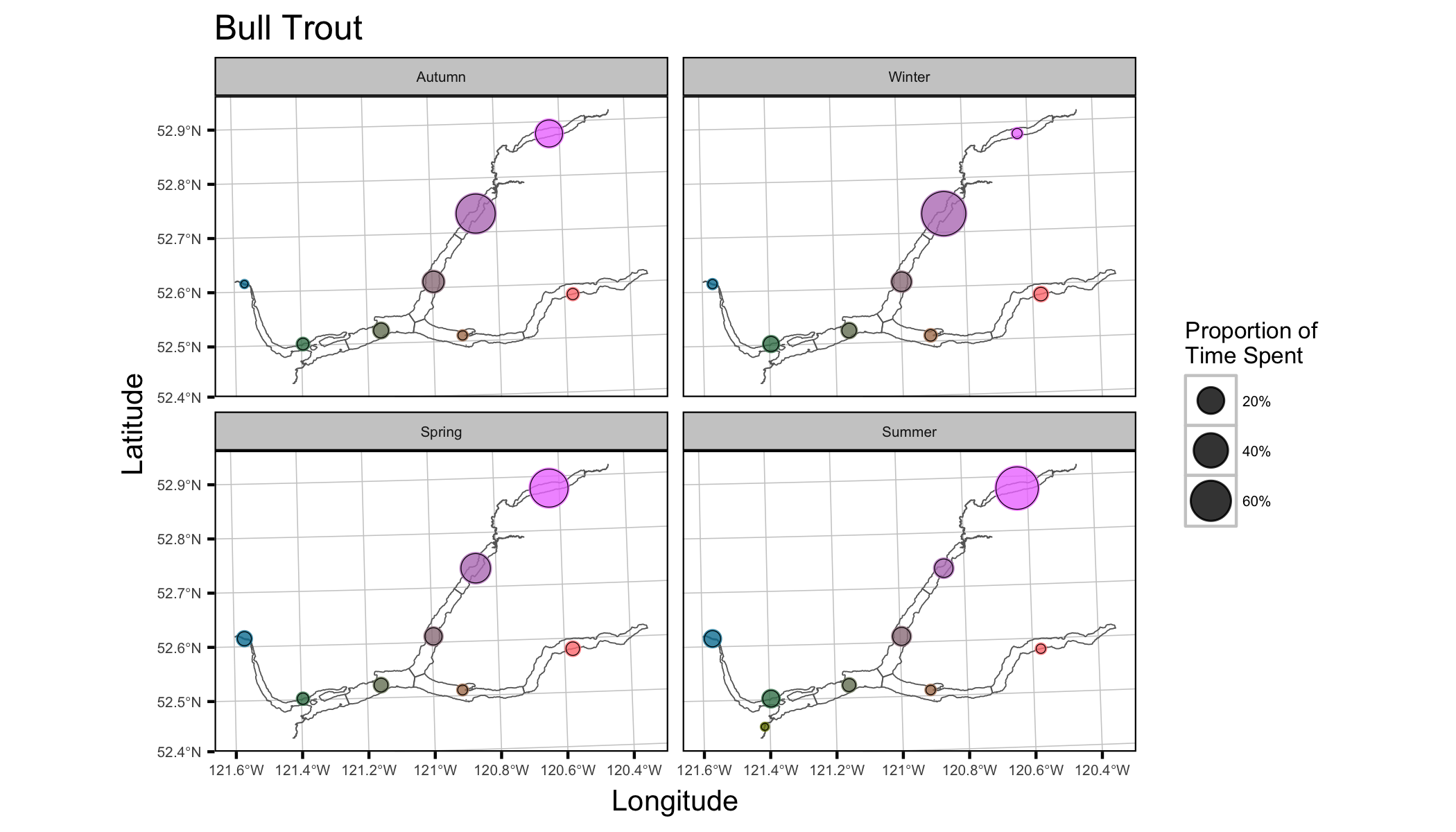Figure 5. Bull Trout percent total duration of stay by section.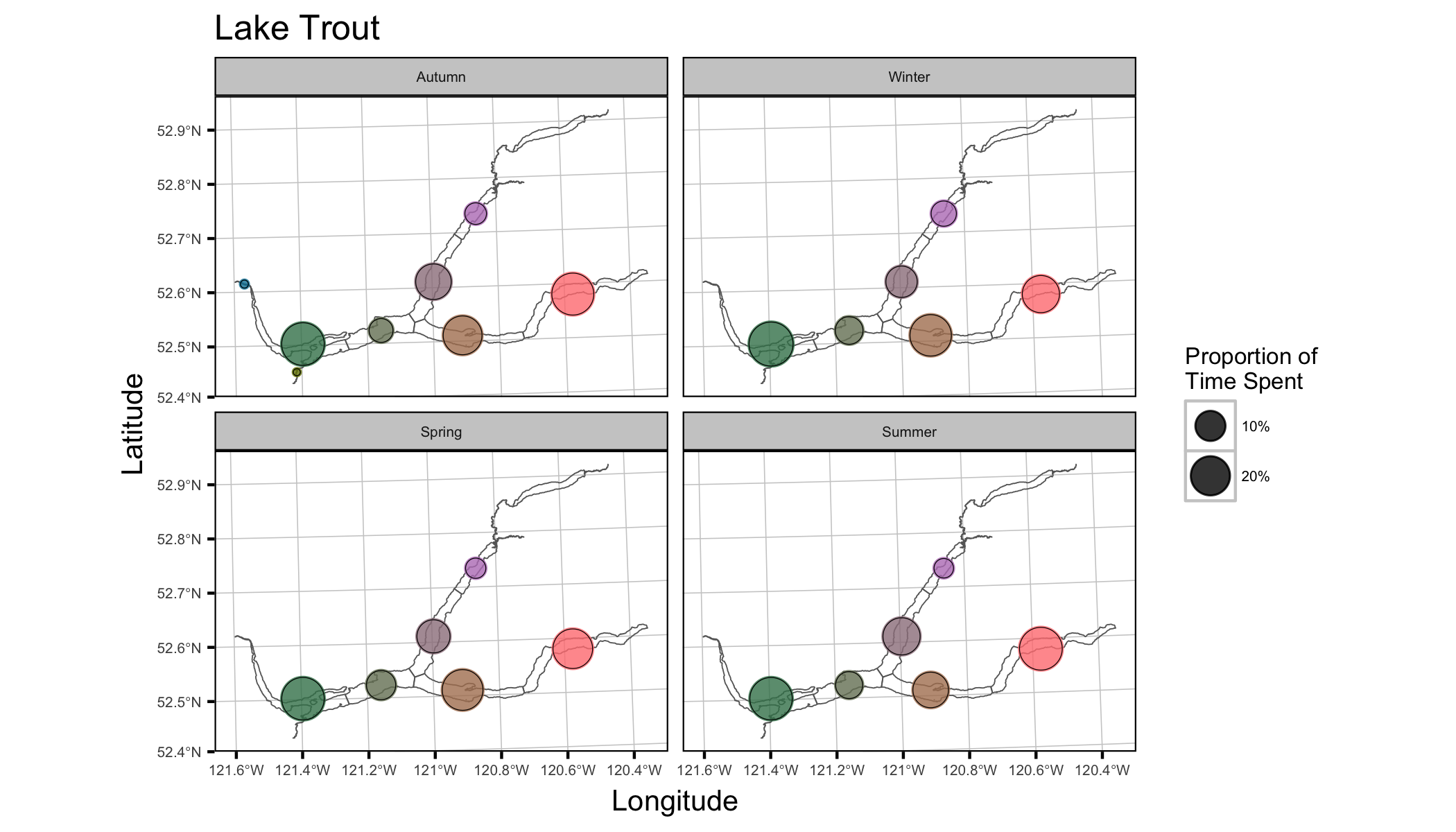Figure 6. Lake Trout percent total duration of stay by section.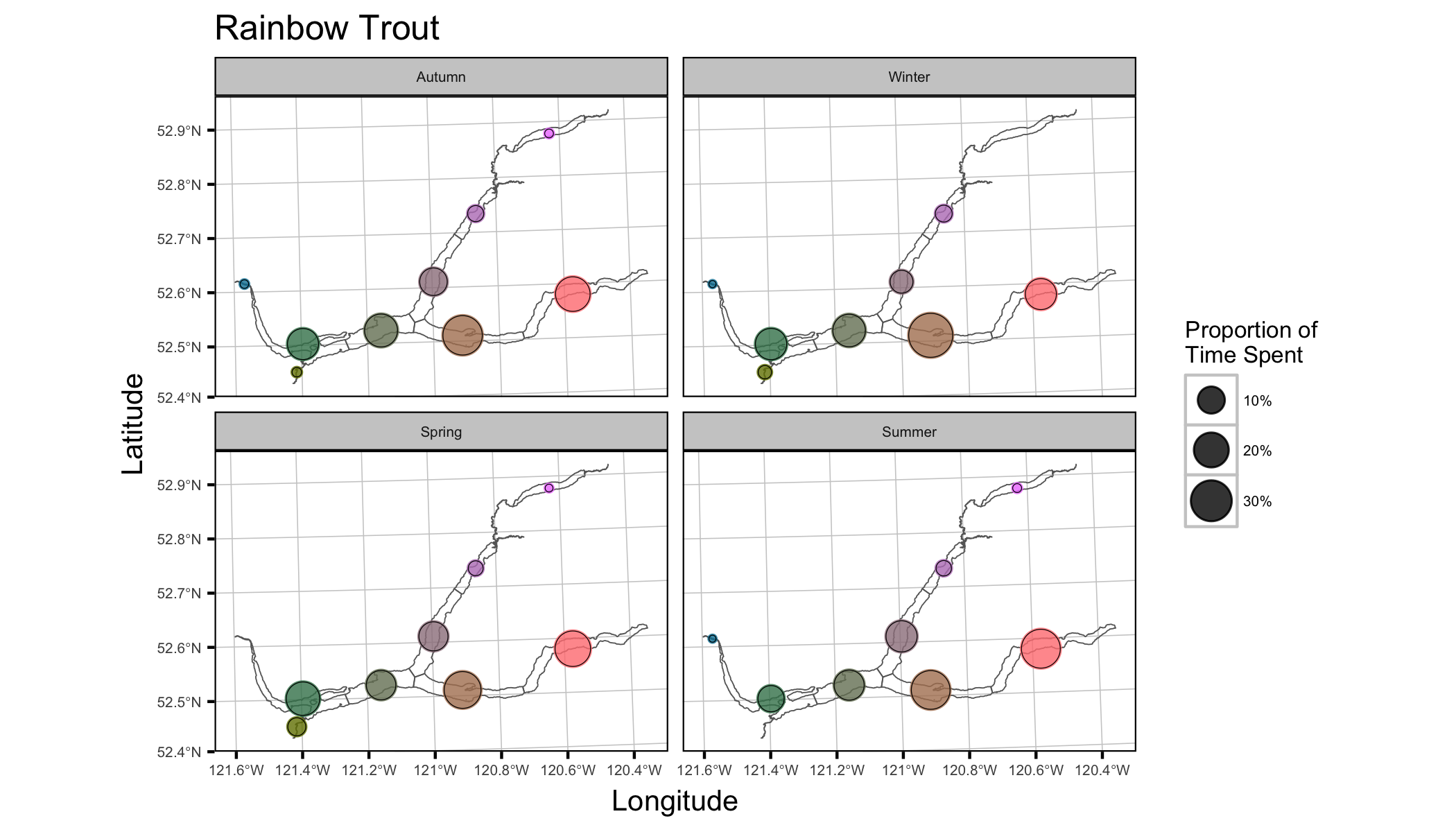Figure 7. Rainbow Trout percent total duration of stay by section.

### Survival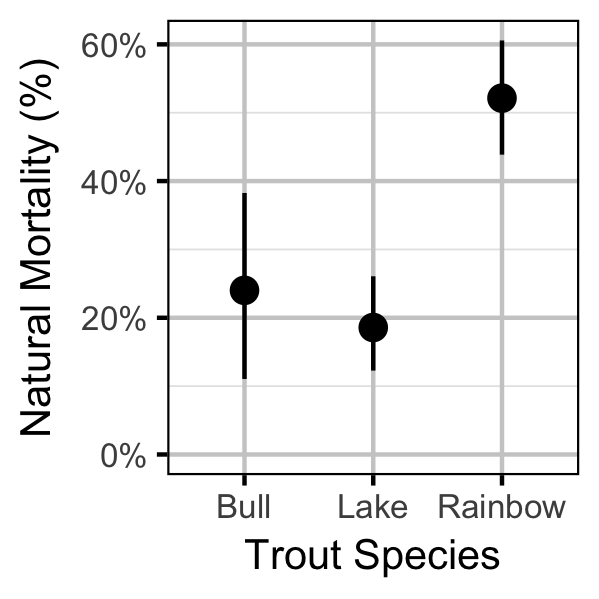Figure 8. The annual interval natural mortality by species.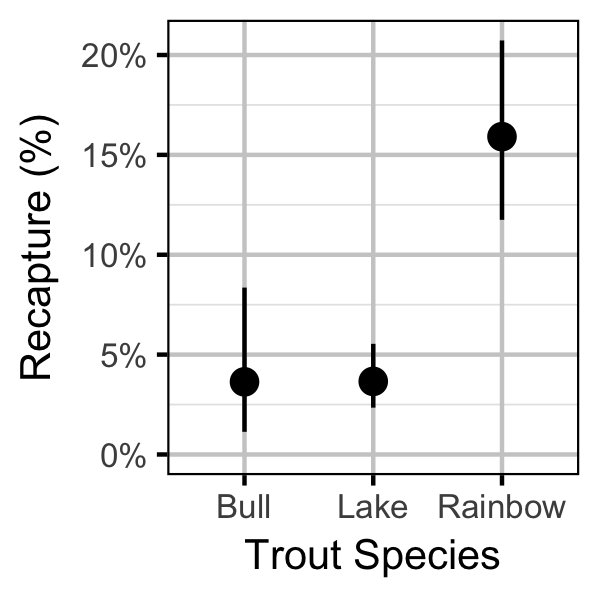Figure 9. The annual interval probability of recapture by species.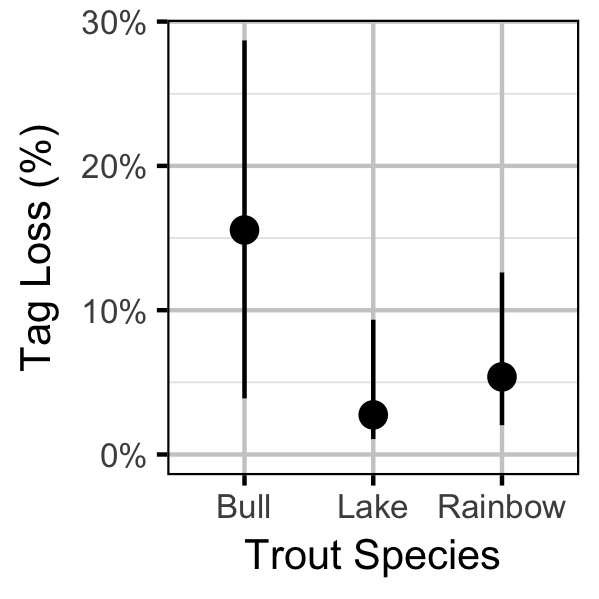Figure 10. The annual probability of T-bar tag loss by species.

## Conclusions

### Recommendations

Recommendations include:

• Develop webpage animating individual fish movements.
• Incorporate recaptures by research crew into survival model.
• Add growth component to survival model to estimate growth parameters and adjust lengths.
• Explore seasonal, annual and length-based variation in natural and fishing mortality.
• Review life-history parameters, in particular $$R_k$$, used in the yield-per-recruit calculations.

## Acknowledgements

The organisations and individuals whose contributions have made this analysis report possible include: